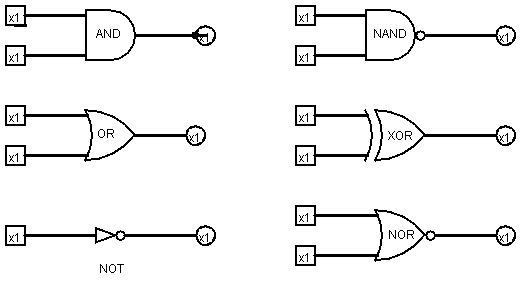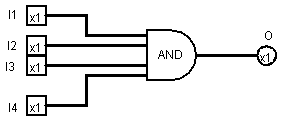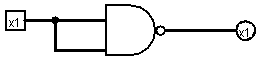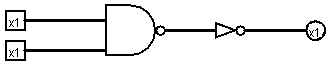# Gates

## Logic Circuit

A logic circuit is a network of logic gates connected by wires.

A logic gate is an input-output device that computes a Boolean operator (AND, OR, NOT, XOR, NAND, NOR, etc.)

Here are the standard symbols for these gates:For example, an AND gate might have four input wires:If all input wires (I1 – I4) carry a high voltage (3 – 5 volts), then the output wire will carry the same high voltage. However, if one or more input wires carry a low voltage (0 – 2 volts), then the output wire will carry a low voltage.

If we identify high voltages with Boolean TRUE and low voltage with FALSE, then this gate computes the Boolean expression:

O = I1 && I2 && I3 && I4

### Question:

How can you build an AND gate with 4 inputs using AND gates that only have two inputs?

## Building NOT, AND, and OR Gates from NAND

We actually only need one type of logic gate: NAND or NOR.

The NAND gate computes the Boolean expression:

O = !(I1 && I2)

Here's the truth table:

I1 I2 NAND
0  0  1
0  1  1
1  0  1
1  1  0

For example, here's how a NOT gate can be built from a NAND gate:In Boolean Algebra we might express this as the law:

!A = A NAND A

Now that we have the NOT gate, we may use it, along with our NAND gate, to build an AND gate:In Boolean Algebra this is just the identity:

A && B = !(A NAND B)

Recall the Boolean laws:

!!A = A

!(A && B) = !A || !B

Combining these laws we get a new law:

A || B = !(!A && !B)

Use this law to build an OR gate from an AND gate and three NOT gates.

Build XOR and NOR from the gates we have so far.

## Transistors

How could we build a NAND gate?

A relay is a switch with two input wires (in and control) and an output wire:When the voltage on the control wire is high, the switch opens and the voltage on the output wire equals 0. If the voltage on the input wire is constantly high, then out is the Boolean complement of control. In other words, the relay becomes a simple NOT gate.

out = !control

Suppose we tie to relays together:Note that only when A = B = 1 are both switches open and therefore the voltage on C will be low. In other words, we have built a NAND gate from relays.

But how do we build a relay?

In a magnetic relay, the control wire activates a magnet which pulls the switch open. A spring closes the switch when the control wire is off.

Unfortunately, a switch in a computer needs to open and close several million times every second. This could never happen if the switch were mechanical.

A semi-conductor is a material that can rapidly alter is ability to conduct electricity when a small current is applied to it. This is the essential technology behind transistors, which are used as high-speed switches in logic gates.